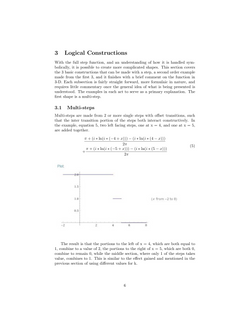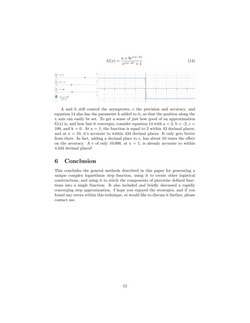## Link to an old commentary trying to work with Fourier Transforms of the Riemann Function

#### A Unique Step Function

This paper discusses a unique complex logarithmic function that can be used as an alternative to piecewise deﬁned step functions such as the Heaviside function. It describes how to create second tier logical functions such as multi-steps, boxcars, and valleys, and it provides a method to stitch the components of piecewise functions into a single function. You can download the pdf or view the paper in the gallery below. If you are having trouble viewing the images, use the pdf instead.## Step Function_01## Step Function_02## Step Function_03## Step Function_04## Step Function_05## Step Function_06## Step Function_07## Step Function_08## Step Function_09## Step Function_10## Step Function_11## Step Function_12

#### Equation Map

The Equation Map is an idea for a project that currently I will never get to pursue, due to the size of the undertaking, and the time constraints it would require. None the less, it's something that will eventually be created by default as society continues to progress. It can be viewed generally in 2 parts. Part 1, the math side, and part 2, the science side. In short, it's a map of equations across fields of study across the circle.

The math side recognizes that based on the 4 basic operations of math: adding, subtraction, multiplication, and division, combined with a small handful of other generic operations: exponentiation, summations, product functions, derivation, integration, and matrix operations, that a large portion, "99% or so," of all possible math formulae can be systematically created using combinatorics on those operations. Such a system would be able to generate all possible formulae arranged by complexity, and would serve as an "Equation Map" of all equations. Visually, this can be thought of by asking for example, where do the equations of general relativity positionally fall in relation to those used in say double helix modeling? This would be helpful such that when an equation was needed for a new or existing model, it would provide a place to start looking for it, or from which to branch out.

The science side begins with the current known given equations of physics, and clusters and links them together based on operators and variable dependencies. For example, all equations that were the sum of 2 constants would have pointers to each other, all those that used the speed of light would be linked, or all equations that used meters would be connected via chains, and so on. From there, one would be able to better investigate which variables were truly independent versus dependent ones, to simplify formulae, as well as be able to start analyzing the overall structure of those formulae clusters. Those groupings of the physics map could then be compared and aligned to the math equation map, resulting in a smaller divide between pure math and physical math applications. This would also facilitate and increase the speed at which unsolved physics and science questions would be solved.

For quick contemporary reference and example of this concept, this is akin to the work of the Wolfram Physics Project, recent work done updating the International System of Units, and work attempted by a Rogue Physics YouTuber by the name of Fractal Woman.

This concept aligns with the Parameter Composite Modeling concept on the Rogue Physics page, and is roughly the infinite parameterization to which it refers.